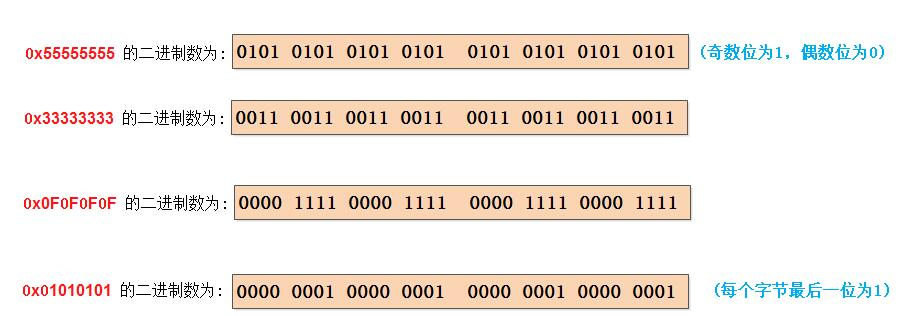## 1. variable-precision SWAR算法

variable-precision SWAR算法通常用于统计一个数组中非0位的数量，数学上被称为计算汉明重量（Hamming Weight)。

### 1.2 算法分析• 步骤1

    i           j         i - j
----------------------------------
0 = 0b00    0 = 0b00    0 = 0b00
1 = 0b01    0 = 0b00    1 = 0b01
2 = 0b10    1 = 0b01    1 = 0b01
3 = 0b11    1 = 0b01    2 = 0b10

• 步骤2

• 步骤3

• 步骤4## 2. Redis中求bit count的实现

Redis中求bit count，用到了查表和variable-precision SWAR两种算法：

• 查表算法使用键长为8位的表，表中记录了从0x00 ~ 0xFF在内的所有二进制位的汉明重量；

• 至于variable-precision SWAR算法方面，Redis会每次处理128个二进制位，这128个bit会通过调用4次32位variable-precision SWAR算法来计算其汉明重量。

[参看]